# 2 3 Atomic Number Mass Number and Isotopes

• Slides: 24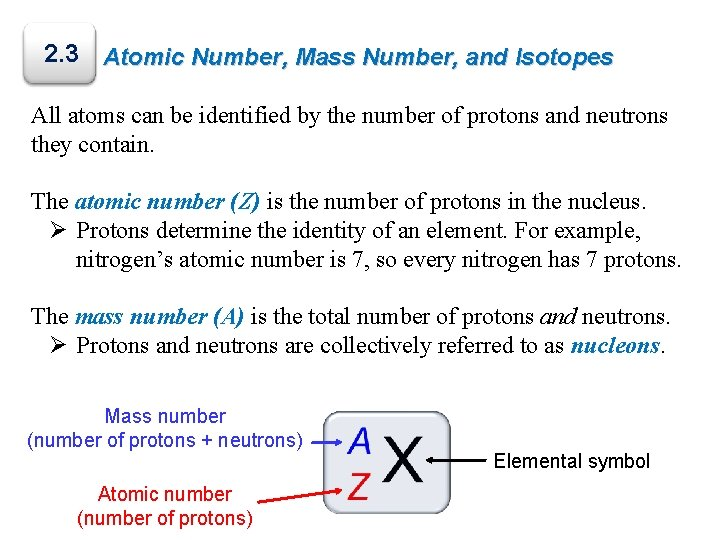2. 3 Atomic Number, Mass Number, and Isotopes All atoms can be identified by the number of protons and neutrons they contain. The atomic number (Z) is the number of protons in the nucleus. Ø Protons determine the identity of an element. For example, nitrogen’s atomic number is 7, so every nitrogen has 7 protons. The mass number (A) is the total number of protons and neutrons. Ø Protons and neutrons are collectively referred to as nucleons. Mass number (number of protons + neutrons) Atomic number (number of protons) Elemental symbolAtomic Number, Mass Number, and Isotopes Most elements have two or more isotopes, atoms that have the same atomic number (Z) but different mass numbers (A). 1 proton 0 neutrons 1 proton 1 neutron 1 proton 2 neutrons Isotopes of the same element exhibit similar chemical properties, forming the same types of compounds and displaying similar reactivities.2. 4 Nuclear Stability The number of protons and neutrons present in an atom determine the stability of the nucleus – will discuss in detail in chapter 20 (CHMY 172) 32. 5 Average Atomic Mass Atomic mass is the mass of an atom in atomic mass units (amu). 1 amu = 1/12 the mass of a carbon-12 atom The average atomic mass on the periodic table represents the average mass of the naturally occurring mixture of isotopes. Isotope Isotopic mass (amu) Natural abundance (%) 12 C 12. 00000 98. 93 13 C 13. 003355 1. 07 Average mass (C) = (0. 9893)(12. 00000 amu) + (0. 0107)(13. 003355 amu) = 12. 01 amu Which isotope has a greater abundance naturally, bromine-79 or bromine-81?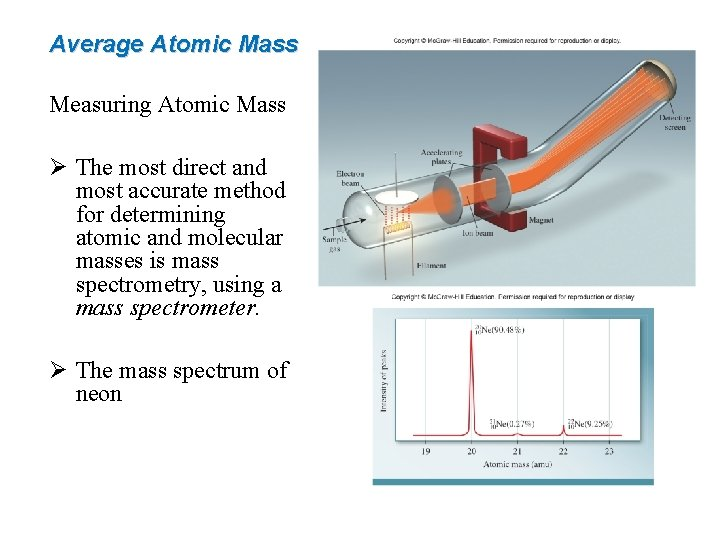Average Atomic Mass Measuring Atomic Mass Ø The most direct and most accurate method for determining atomic and molecular masses is mass spectrometry, using a mass spectrometer. Ø The mass spectrum of neonWorked Example 2. 1 Determine the numbers of protons, neutrons, and electrons in each of the 35 41 37 following species: (a) 17 Cl, (b) Cl, (c) K, and (d) carbon-14. Strategy Recall the superscript denotes the mass number (A) and the subscript denotes the atomic number (Z). If no subscript is shown, the atomic number can be deduced from the elemental symbol or name. There is no charge shown on these atoms. • https: //phet. colorado. edu/en/simulation/build-an-atom Text Practice: 2. 14 2. 18 a)2. 6 The Periodic Table The periodic table is a chart in which elements having similar chemical and physical properties are grouped together.The Periodic Table Elements are arranged in periods, horizontal rows, in order of increasing atomic number.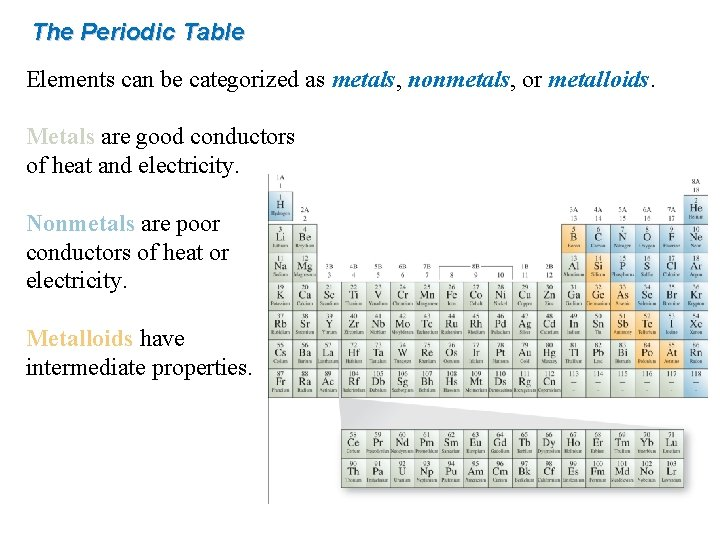The Periodic Table Elements can be categorized as metals, nonmetals, or metalloids. Metals are good conductors of heat and electricity. Nonmetals are poor conductors of heat or electricity. Metalloids have intermediate properties.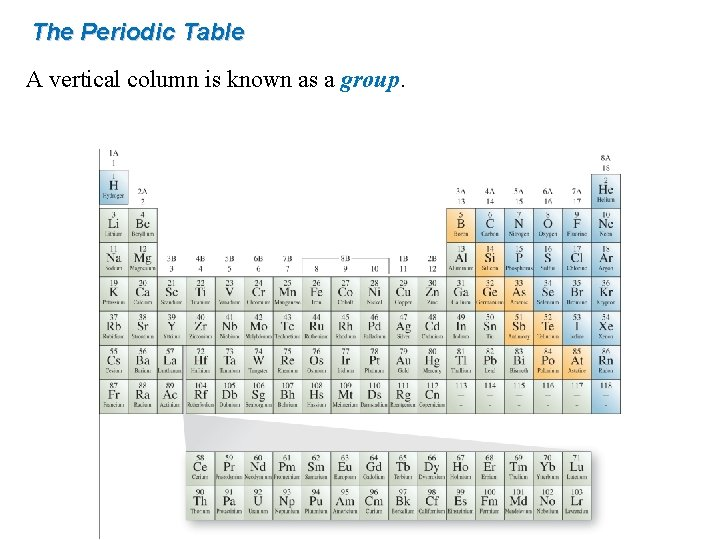The Periodic Table A vertical column is known as a group.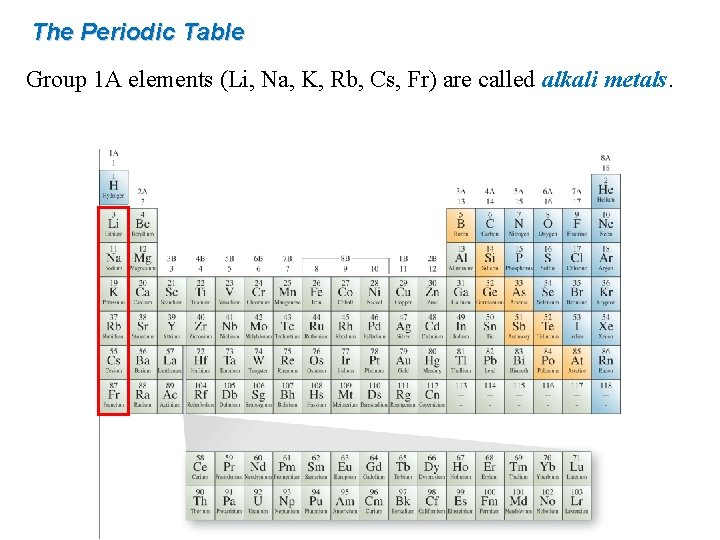The Periodic Table Group 1 A elements (Li, Na, K, Rb, Cs, Fr) are called alkali metals.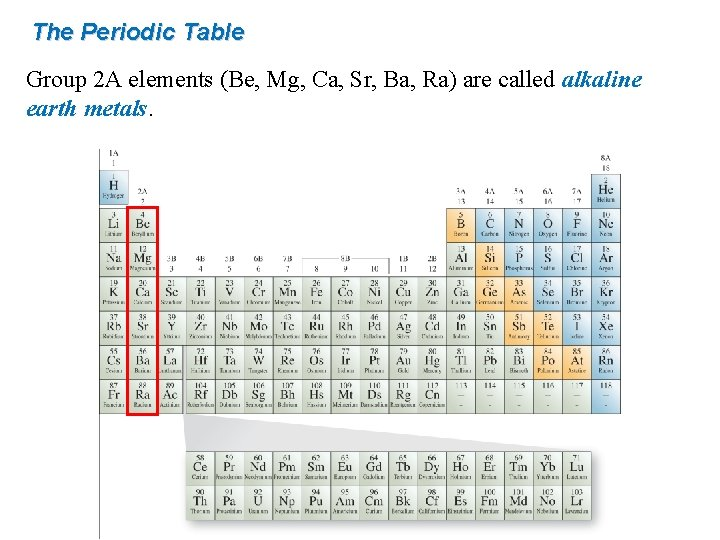The Periodic Table Group 2 A elements (Be, Mg, Ca, Sr, Ba, Ra) are called alkaline earth metals.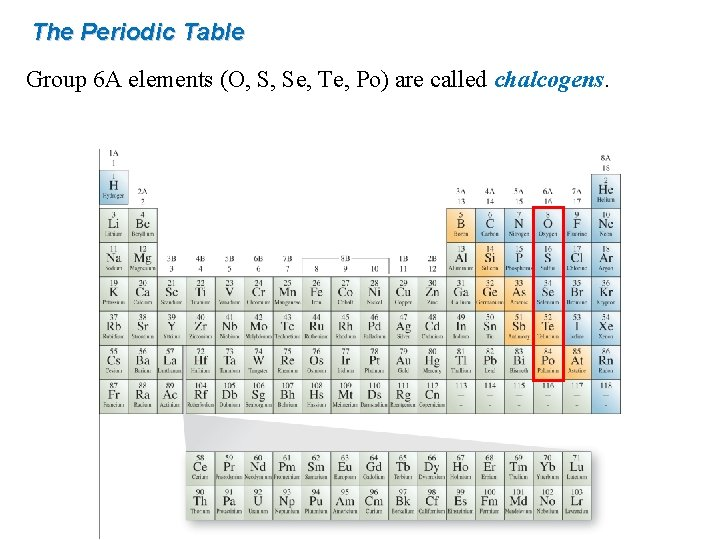The Periodic Table Group 6 A elements (O, S, Se, Te, Po) are called chalcogens.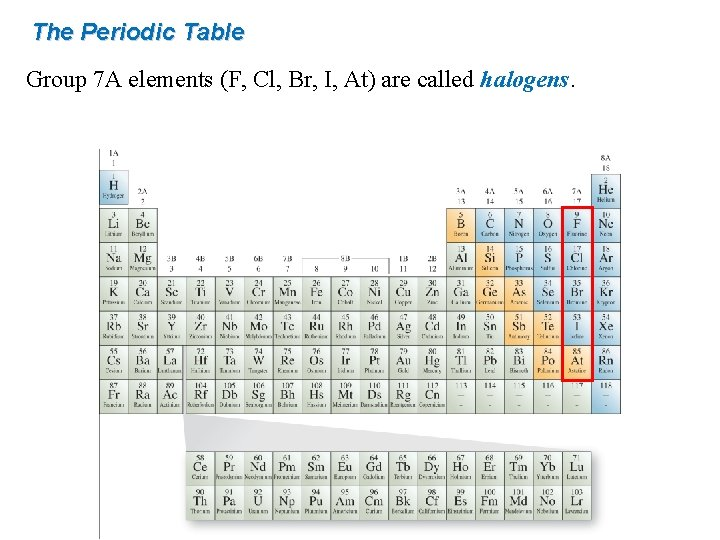The Periodic Table Group 7 A elements (F, Cl, Br, I, At) are called halogens.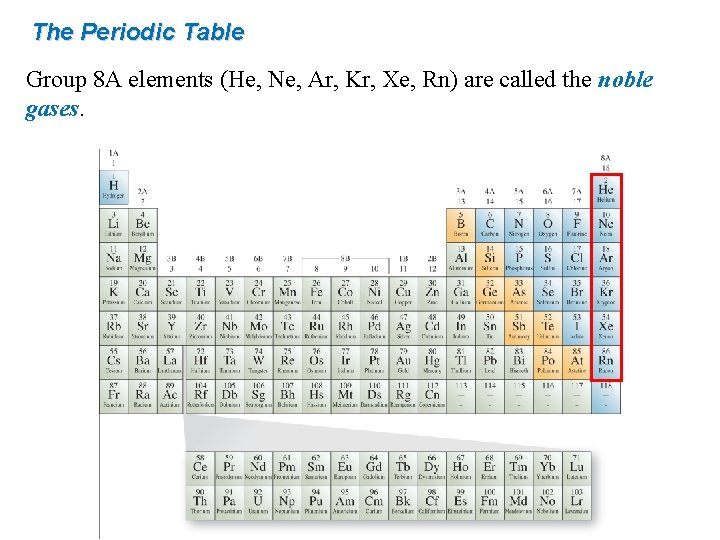The Periodic Table Group 8 A elements (He, Ne, Ar, Kr, Xe, Rn) are called the noble gases.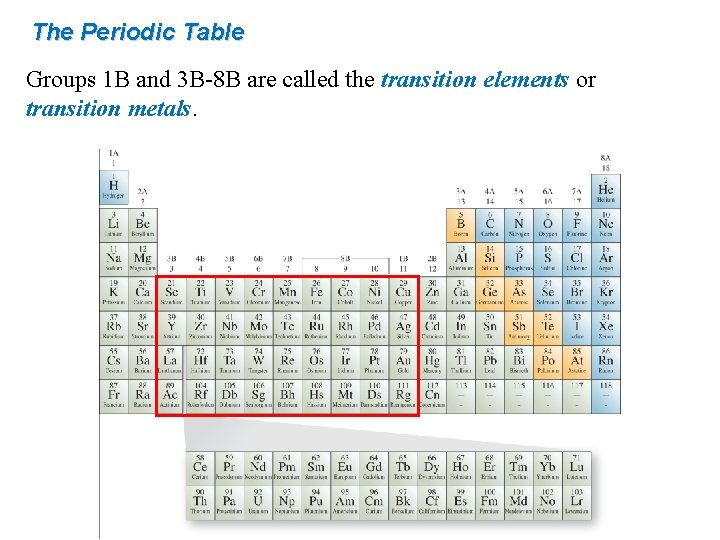The Periodic Table Groups 1 B and 3 B-8 B are called the transition elements or transition metals.Chemistry: Atoms First Second Edition Julia Burdge & Jason Overby Chapter 3 Quantum Theory and the Electronic Structure of Atoms M. Stacey Thomson Pasco-Hernando State College Copyright (c) The Mc. Graw-Hill Companies, Inc. Permission required for reproduction or display.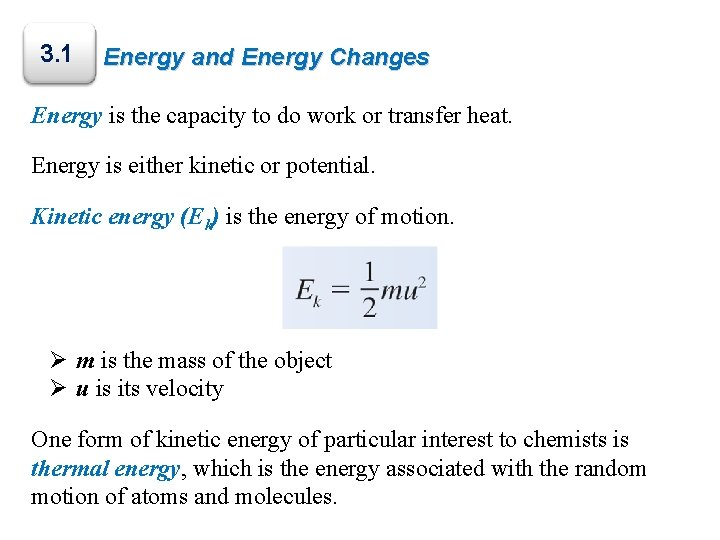3. 1 Energy and Energy Changes Energy is the capacity to do work or transfer heat. Energy is either kinetic or potential. Kinetic energy (Ek) is the energy of motion. Ø m is the mass of the object Ø u is its velocity One form of kinetic energy of particular interest to chemists is thermal energy, which is the energy associated with the random motion of atoms and molecules.Forms of Energy Potential energy is the energy possessed by an object by virtue of its position or stability. There are two forms of potential energy of great interest to chemists: Ø Chemical energy is energy stored within the structural units of chemical substances. Ø Electrostatic energy is potential energy that results from the interaction of charged particles. Q 1 and Q 2 represent two charges separated by the distance, d.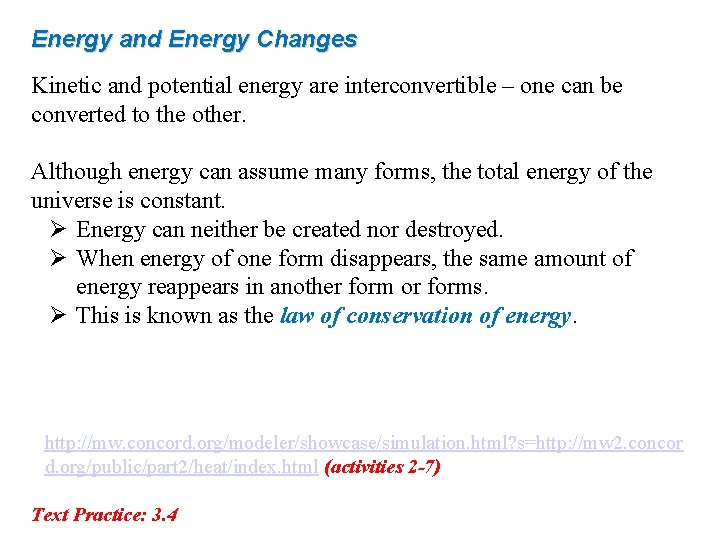Energy and Energy Changes Kinetic and potential energy are interconvertible – one can be converted to the other. Although energy can assume many forms, the total energy of the universe is constant. Ø Energy can neither be created nor destroyed. Ø When energy of one form disappears, the same amount of energy reappears in another form or forms. Ø This is known as the law of conservation of energy. • http: //mw. concord. org/modeler/showcase/simulation. html? s=http: //mw 2. concor d. org/public/part 2/heat/index. html (activities 2 -7) Text Practice: 3. 4Units of Energy The SI unit of energy is the joule (J), named for the English physicist James Joule. It is the amount of energy possessed by a 2 -kg mass moving at a speed of 1 m/s. Ek = ½ mu 2 = ½(2 kg)(1 m/s)2 = 1 kg∙m 2/s 2 = 1 J The joule can also be defined as the amount of energy exerted when a force of 1 newton (N) is applied over a distance of 1 meter. 1 J=1 N·m Because the magnitude of a joule is so small, we often express large amounts of energy using the unit kilojoule (k. J). 1 k. J = 1000 JStudy Guide for sections 2. 3 -2. 6, 3. 1 DAY 4, Terms to know: Sections 2. 3 -2. 6, 3. 1 atomic number, mass number, isotope, average mass, isotopic natural abundance, periodic table, halogens, noble gasses, transition metals, energy, kinetic energy, thermal energy, potential energy, chemical energy, electrostatic energy, law of conservation of energy, joule DAY 4, Specific outcomes and skills that may be tested on exam 1: Sections 2. 3 -2. 6, 3. 1 • Be able to use elemental symbols to determine how many protons, neutrons, and electrons are present in an atom • Given a specific number of protons, neutrons, and electrons, be able to give a complete elemental symbol including element, mass, and charge • Given isotopes and their natural abundance, be able to calculate the average atomic mass • Given an average atomic mass and two or three isotopes, be able to describe the isotopes relative abundances • Be able to describe possible transformations from one form of energy to another • Given a process that involve a transfer of energy, be able to describe what forms of energy exist before and after the process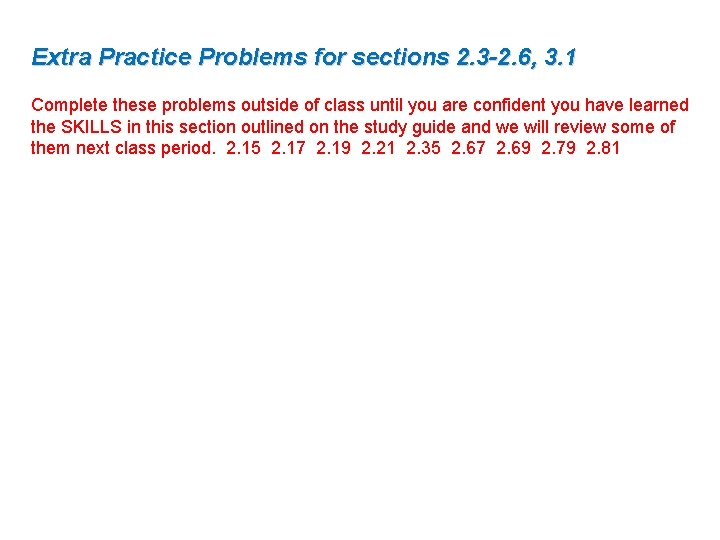Extra Practice Problems for sections 2. 3 -2. 6, 3. 1 Complete these problems outside of class until you are confident you have learned the SKILLS in this section outlined on the study guide and we will review some of them next class period. 2. 15 2. 17 2. 19 2. 21 2. 35 2. 67 2. 69 2. 79 2. 81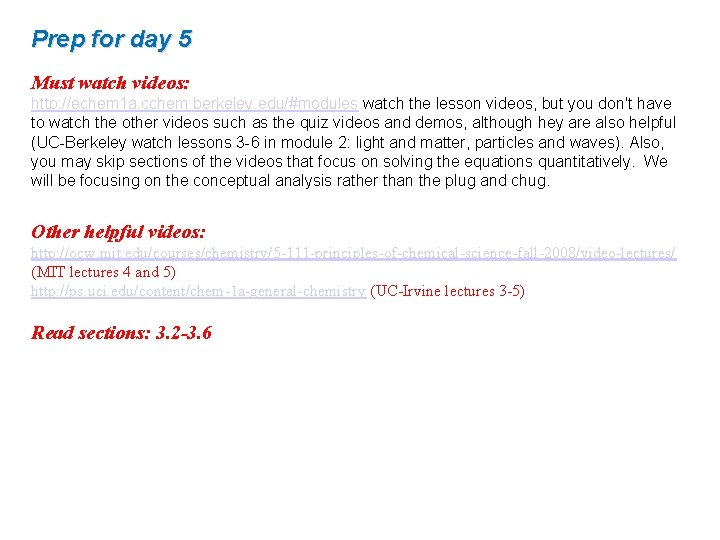Prep for day 5 Must watch videos: http: //echem 1 a. cchem. berkeley. edu/#modules watch the lesson videos, but you don't have to watch the other videos such as the quiz videos and demos, although hey are also helpful (UC-Berkeley watch lessons 3 -6 in module 2: light and matter, particles and waves). Also, you may skip sections of the videos that focus on solving the equations quantitatively. We will be focusing on the conceptual analysis rather than the plug and chug. Other helpful videos: http: //ocw. mit. edu/courses/chemistry/5 -111 -principles-of-chemical-science-fall-2008/video-lectures/ (MIT lectures 4 and 5) http: //ps. uci. edu/content/chem-1 a-general-chemistry (UC-Irvine lectures 3 -5) Read sections: 3. 2 -3. 6